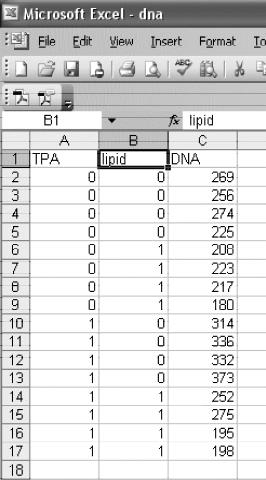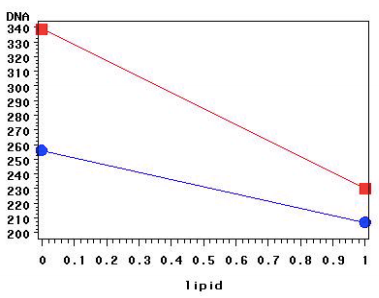## Plot a by b means using SAS UE

Hello,

I'm trying to run an sgplot procedure using SAS UE. The purpose is to plot a variable by another variable means. I have the procedure I am trying to follow that uses gplot (which does not work for SAS UE). I'm not sure what the equivalent would be when using SAS UE. I appreciate any help regarding this!

Procedure given (cannot be used for SAS UE):

proc gplot data=clinical;
axis1 length=4 in;
axis2 length=6 in;
plot dna*lipid=tpa / vaxis=axis1 haxis=axis2;
symbol1 v=J f=special h=3 i=join color=blue;
symbol2 v=K f=special h=3 i=join color=red;
run;

Data:Final plot (tpa by lipid means, goal for SAS UE procedure not yet gotten):1 ACCEPTED SOLUTION

Accepted Solutions

## Re: Plot a by b means using SAS UE

This is called an "Interaction Plot" and it is produced automatically when you perform a two-way analysis of mean with an interaction term:

``````data dna;
input TPA lipid DNA;
datalines;
0 0 269
0 0 256
0 0 274
0 0 225
0 1 208
0 1 223
0 1 217
0 1 180
1 0 314
1 0 336
1 0 332
1 0 373
1 1 252
1 1 276
1 1 195
1 1 198
;

proc glm data=DNA plots=IntPlot;
class TPA lipid;
model DNA = TPA | lipid;
run;
``````

If you don't want to see the observed values, you can write the model to an item store and use PROC PLM to create an effect plot:

``````proc glm data=DNA;
class TPA lipid;
model DNA = TPA | lipid;
store GlmModel;
run;

proc plm restore=GLMModel;
effectplot;
run;
``````
2 REPLIES 2PGStats
Opal | Level 21

## Re: Plot a by b means using SAS UE

Something like this should do:

``````proc sql;
create table graph as
select
tpa,
mean(lipid) as lipid,
mean(dna) as dna
from clinical
group by tpa;
quit;

ods graphics / height=4in width=6in;
proc sgplot data=graph noautolegend;
styleattrs
DATACONTRASTCOLORS=(blue red)
DATASYMBOLS=(squarefilled circlefilled);
series y=dna x=lipid / group=tpa
markers markerattrs=(size=8)
lineattrs=(pattern=solid);
run;``````
PG

## Re: Plot a by b means using SAS UE

This is called an "Interaction Plot" and it is produced automatically when you perform a two-way analysis of mean with an interaction term:

``````data dna;
input TPA lipid DNA;
datalines;
0 0 269
0 0 256
0 0 274
0 0 225
0 1 208
0 1 223
0 1 217
0 1 180
1 0 314
1 0 336
1 0 332
1 0 373
1 1 252
1 1 276
1 1 195
1 1 198
;

proc glm data=DNA plots=IntPlot;
class TPA lipid;
model DNA = TPA | lipid;
run;
``````

If you don't want to see the observed values, you can write the model to an item store and use PROC PLM to create an effect plot:

``````proc glm data=DNA;
class TPA lipid;
model DNA = TPA | lipid;
store GlmModel;
run;

proc plm restore=GLMModel;
effectplot;
run;
``````
Discussion stats
• 2 replies
• 327 views
• 6 likes
• 3 in conversation